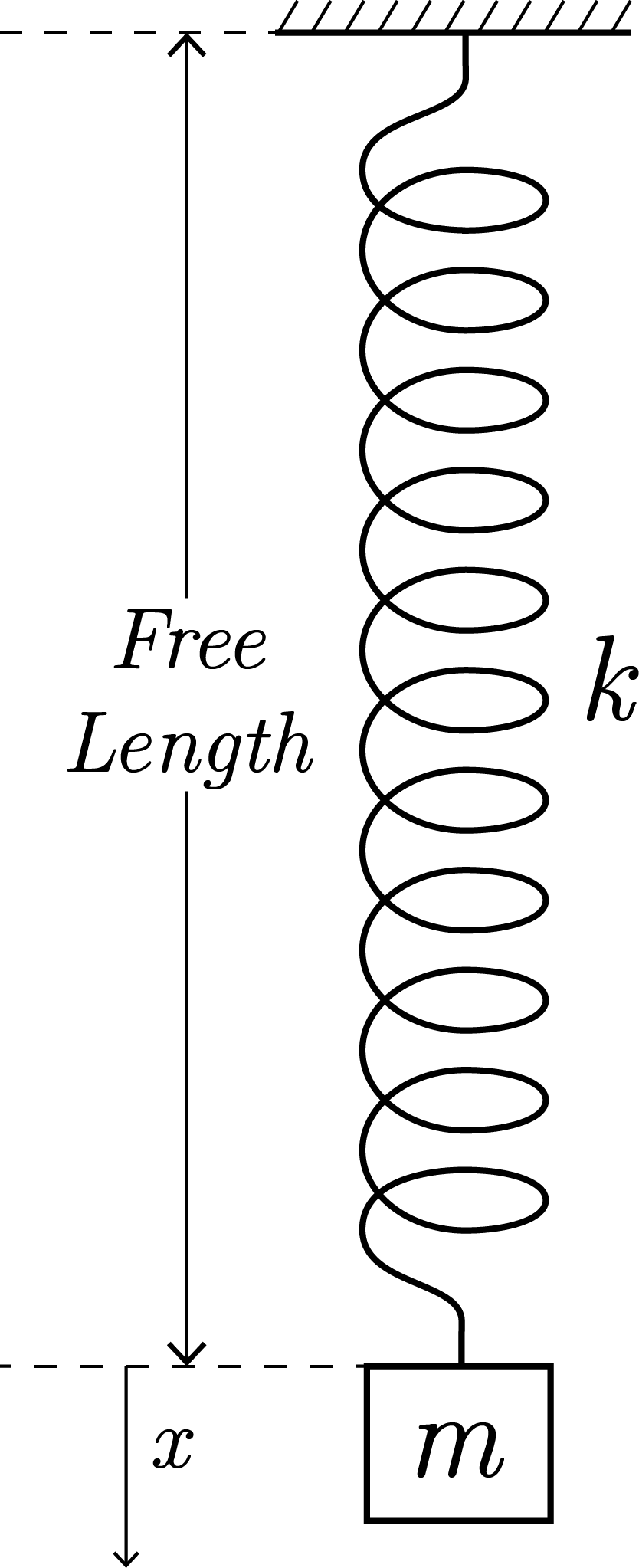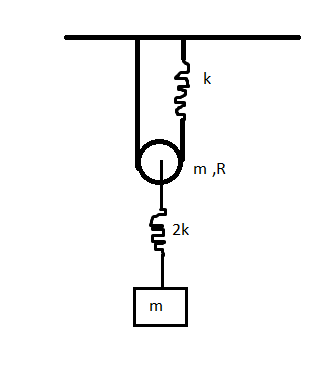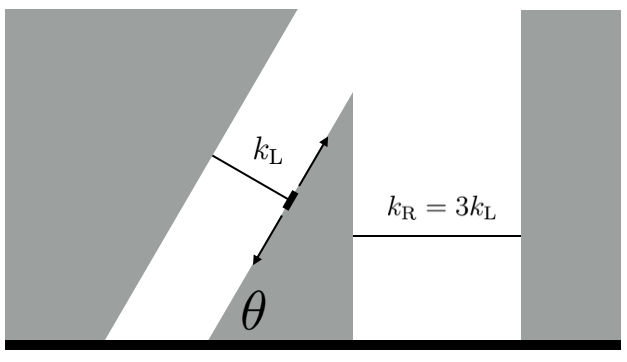Classical Mechanics

# Simple Harmonic Motion: Level 4-5 Challenges

Consider the earth as a uniform sphere of mass $M$ And Radius $R$. Imagine a straight smooth tunnel made through the earth which connects any two points on its surface (The two points are not diametrically opposite). Determine the time that a particle would take to go from one end to the other through the tunnel. If Your answer is $T s$, then find the value of $\frac{T}{100}$ to the nearest integer.

Details And Assumptions:

• Consider only gravitation force due to earth be acting on the particle during its motion along the tunnel.

• Take acceleration due to gravity on surface of Earth = $10ms^{-2}$

• Radius of Earth = $6400 km$.

This is an entry for the problem writing party.A block of mass $m$ is gently attached to the spring and released at time $t=0$, when the spring has its free length. During subsequent motion of the block, the displacement of the block $x$ with respect to time $t$ is considered. Find $\displaystyle\int_0^3 x ~\mathrm{d}t$

Details and Assumptions

• Neglect air resistance.
• $k$ is the spring constant.
• $m = 10\text{ kg},k = 34 \text{ Nm}^{-1}, g = 9.8 \text{ ms}^{-2}$

### This Question is not Original

A mass is subjected to a force $F = (at - bx) \text{ along the } x\text{-axis}$ Initially the mass lies at the origin at rest. In the definition of force (given) $x$ refers to the $x$-coordinate of the mass and t refers to the time elapsed.

Find the x - coordinate of the mass after a time of 4 seconds.

Assumptions
1) All the values are in SI units.
2) Take the mass = 1 kg, a = 1 N/s, b = 1 N/mIf the time period of SHM of rectangular block can be represented as $T=2\pi \sqrt{\frac{m(\sqrt a +b)}{ck}}$

Find the value of $a+b+c$.

Details and Assumptions:

• Treat the pulley as a disc.

• The block and pulley are oscillating in the same phase with the same frequency.

• Pulley has sufficient friction for Pure Rolling and mass=m & strings are light and inextensible.

• Gravity is present.

• Zig-zag lines in fig represents spring of spring constant $k$ and $2k$ as shown.

• a is a square-free integer and the fraction is in simplest form.

###### Try its different variants - medium and easyA smooth wedge of mass $m$ and angle of inclination $\theta = \SI{60}{\degree}$ is attached to two springs of spring constant $k_\textrm{L}$ on the left, and $k_\textrm{R} = 3k_\textrm{L}$ on the right. The wedge rests on a smooth frictionless plane. Find the period of oscillation of the wedge in seconds.

Details and Assumptions:

• The springs are perpendicular to the respective sides they are facing.
• The spring on the left is constrained to compress and extend along its length. It is attached to the wedge by a frictionless roller that can move along the hypotenuse.
• $m=\SI{3}{\kilo\gram}$
• $k_\textrm{L}= \frac18 \si[per-mode=symbol]{\newton\per\meter}$.
×